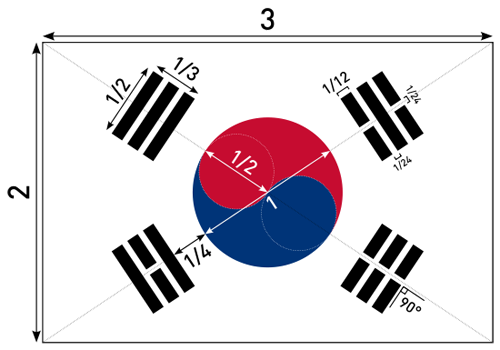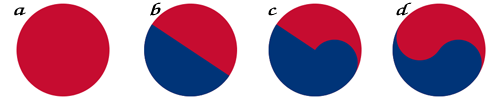# How to Draw the South Korean Flag

28 Nov

This is one a series of posts on how to draw world flags. Finding the specifications for these flags, and attempting to reproduce the flag perfectly, according to the specifications, is a great way to teach yourself basic graphics programming.

Here’s my sketch of the flag of South Korea.

As usual, I found the specification on Wikipedia. It contains the following helpful construction sheet:This flag has a width which is 3/2 the height of the flag. It consists of two types of figures, the taegeuk in the center, and the trigrams that surround it.

We start by filling the display area with white.

```background(whiteColor);
```

I’ve drawn the taegeuk as a series of circles. First I position to the center (via translate) and rotate so that the figure will be aligned with the diagonal of the flag. We push the transformation matrix so we can restore it later.

```float rotAngle = atan2(height/2, width/2);
pushMatrix();
translate(width/2, height/2);
rotate(rotAngle);
```

Then we draw the taegeuk in four steps:```// Step A
fill(redColor);
arc(0,0,circWidth, circWidth, 0, TWO_PI);

// Step B
fill(blueColor);
arc(0,0,circWidth, circWidth, 0, PI);

// Step C
ellipse(circWidth/4,0, circWidth/2, circWidth/2);

// Step D
fill(redColor);
ellipse(-circWidth/4,0, circWidth/2, circWidth/2);
popMatrix();
```

Finally we are ready to draw the trigrams. I used an old hacker trick and represent the trigrams patterns as 4 integers.

// Bit patterns on trigrams
int[] trigrams = {0,5,7,2};

The 1s and 0s in the binary representation of those numbers will be used to determine if the the stripes are solid, or have a notch in the middle. Here’s the code that draws the trigrams:

``` noStroke();
for (int t = 0; t < 4; ++t) {
pushMatrix();
translate(width/2, height/2);
switch (t) {
case 0:  rotate(rotAngle);      break;
case 1:  rotate(PI-rotAngle);   break;
case 2:  rotate(PI+rotAngle);   break;
case 3:  rotate(-rotAngle);     break;
}
for (int b = 0; b < 3; ++b) {
float x1 = circWidth/2 + triBase + barDist*b;
float y1= -circWidth/4;
fill(blackColor);
rect(x1,y1, barHeight, triWidth);
if ((trigrams[t] & (1 << b)) == 0)
{
// white block
fill(whiteColor);
rect(x1-2,-circWidth/48, barHeight+4, circWidth/24);
}
}
popMatrix();
}
```

The tricky part is this line, where I'm looking for 0 bits:

```    if ((trigrams[t] & (1 << b)) == 0)
```

trigrams[t] is the integer corresponding to the trigram I'm drawing. b is an integer which represents the stripe I am drawing (0,1, or 2). I shift a 1 by that amount (1 << b) to determine the bit position to test. Then I use the bitwise AND (&) operator to test that bit. If the result is 0, we have a zero in that bit position, and I draw a white notch in the center of the bar.

Tags: , , ,

### One Response to “How to Draw the South Korean Flag”

1.kelley April 30, 2012 at 3:14 pm #

very artsy and fun to draw